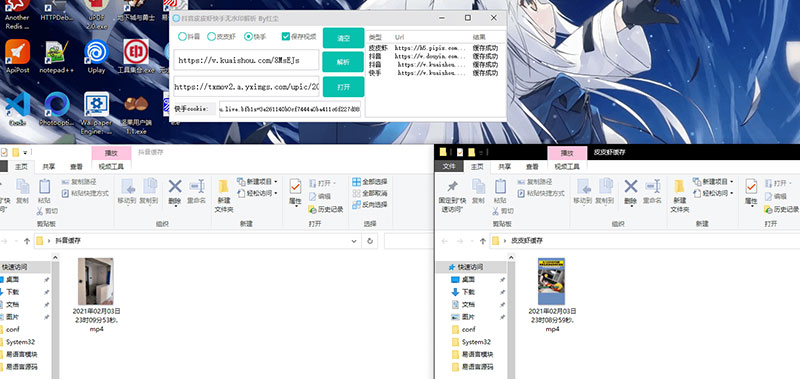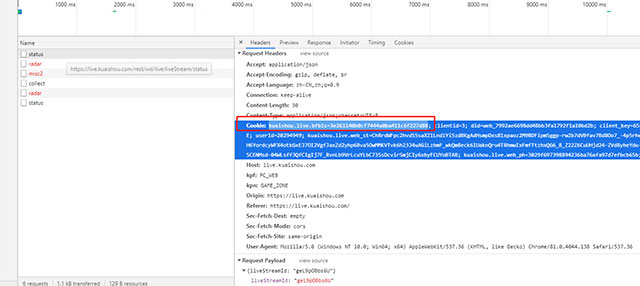### **一、准备**

sublime text 3、360浏览器、Python 3.6运行环境

### **三、操作实践**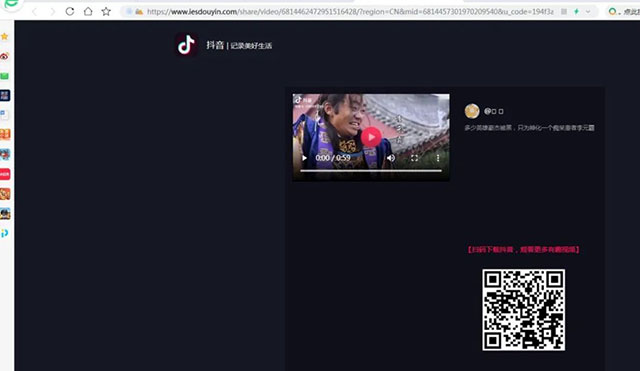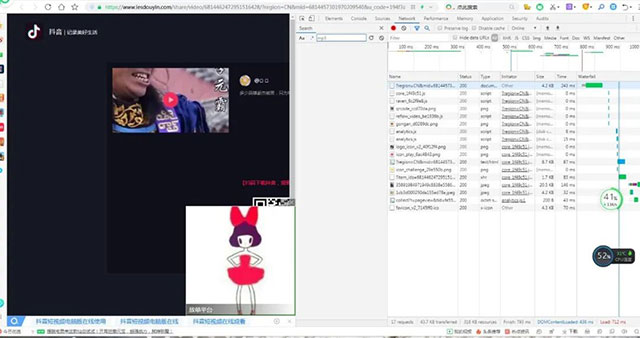class page: def __init__(self): self.root= tk.Tk() #初始化窗口 self.root.title(‘抖音无水印视频下载v1.0′) #窗口名称 self.root.geometry(“700×700”) #设置窗口大小 #设置窗口是否可变，宽不可变，高可变，默认为True self.root.resizable(width=True,height=True) #创建标签，文字，背景颜色，字体（颜色，大小），标签的高和宽 self.label1 =tk.Label(self.root,text=’抖音地址：’,font=(‘宋体’,10),width=12,height=2) #创建输入框，标签高度，字体大小颜色，内容显示方式 self.e1 = tk.Entry(self.root,width=30,show=None, font=(‘Arial’, 12)) self.label2 =tk.Label(self.root,text=’文件名：’,font=(‘宋体’,10),width=12,height=2) #创建输入框，标签高度，字体大小颜色，内容显示方式 self.e2 = tk.Entry(self.root,width=30,show=None, font=(‘Arial’, 12)) #创建按钮 内容 宽高 按钮绑定事件 self.b1 = tk.Button(self.root, text=’无水印下载’, width=8,height=1,command=self.download) self.b2 = tk.Button(self.root, text=’清空内容’, width=8,height=1,command=self.close) self.photo=tk.PhotoImage(file = ‘111.gif’) self.im=tk.Label(self.root, image = self.photo) self.photo1=tk.PhotoImage(file = ‘222.gif’) self.im1=tk.Label(self.root, image = self.photo1) self.dashang=tk.Label(self.root,bg=’gray’,fg=’blue’,font=(‘宋体’,12),text=’感谢各位的支持，觉得小弟写的不错欢迎打赏，以便于我日后分享更多的精彩作品’) #将所有部件添加到界面中 self.label1.place(x=140,y=30,anchor=’nw’) self.e1.place(x=210,y=32,anchor=’nw’) self.b2.place(x=500,y=40,anchor=’nw’) self.label2.place(x=144,y=60,anchor=’nw’) self.e2.place(x=210,y=62,anchor=’nw’) self.b1.place(x=230,y=110,anchor=’nw’) self.dashang.place(x=60,y=160) self.im.place(x=10,y=200,width=202,height=313,anchor=’nw’) self.im1.place(x=300,y=200,width=202,height=313,anchor=’nw’) self.b3=tk.Button(self.root,text=’技术博客，点我直达’,command=self.refer) self.b3.place(x=200,y=530,width=140,height=40,anchor=’nw’) self.root.mainloop()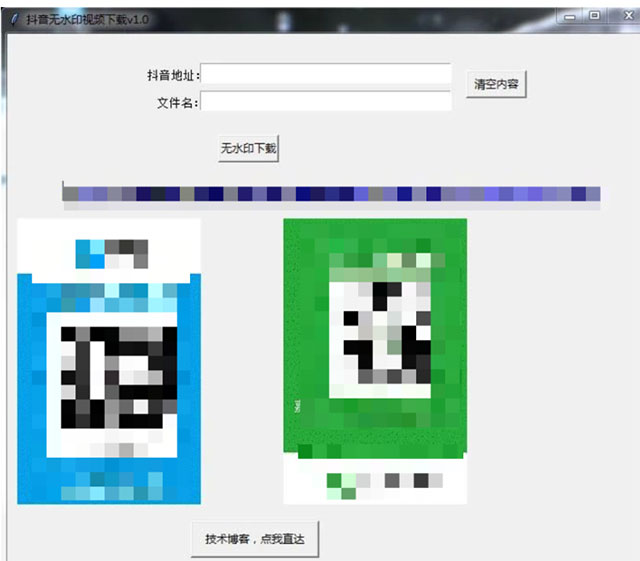def download(self): url=’http://www.zimo.wiki:8080/douyin-video-crawler/api/analysis?url=’+self.e1.get() try: rep=requests.get(url,timeout=5) result=rep.text res=eval(result) if res.get(‘msg’)==’analysis success’: down_url=res.get(‘url’) data=requests.get(down_url,stream=True,timeout=4) with open(‘{}.mp4′.format(self.e2.get()),’wb’) as f: f.write(data.content) else: msg.showwarning(‘notice’,’URL Format Error!’) except: return

http://www.zimo.wiki:8080/douyin-video-crawler/api/analysis?url=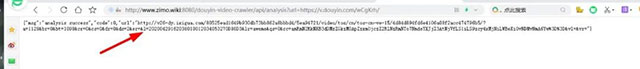def close(self): #关闭 self.e1.delete(0,’end’) self.e2.delete(0,’end’) def refer(self): #跳转 wb.open(‘https://url.ms/lypks’)

page()